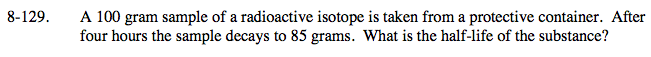### Home > PC > Chapter 8 > Lesson 8.2.4 > Problem8-129

8-129.Substitute the initial amount 100 for 'a'. Substitute 85 for 'y' and 4 for 't'.

Solve for k.

Since we want to find how long it takes for half to decay, solve: 1 = 2e−0.0406t
This indicates an amount of 2 was reduced to 1.

y = aekt

85 = 100e4k

k = −0.0406

t = 17.06 hours